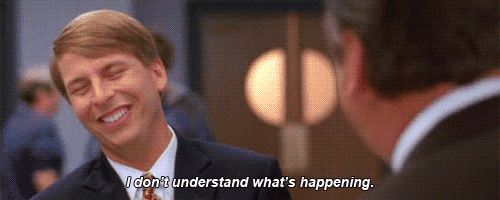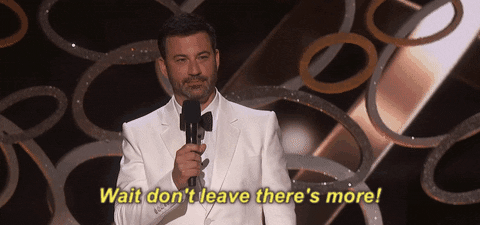## DEV Community is a community of 552,031 amazing developers

We're a place where coders share, stay up-to-date and grow their careers.# x-Bit Integers: Solving With A RangeAidi Rivera Updated on ・5 min read

In my previous article I gave a short introduction to what x-bit and binary integers are for those who might not have a computer science background. I sorta knew what binary numbers were and the whats and whys but I didn't really know. Not enough to do anything with them, at least.

So this time I'll cover how to deal with problems that are asking to account for 8, 16, 32, or 64-bit integers.

## Max and Min Range

The most common way I've seen x-bit integers used in algorithms is as a note on the bit range of integers that the function will accept or return. It might look something like this:

### the problem

Given a 32-bit integer, reverse digits of an integer.

The first time I saw this, I completely ignored it because - wut?

But as soon as I did some research I realized it's all about the size of the integer. In this case, the integer the function can return will be a 32-bit integer. That means any decimal number whose binary equivalent is larger than 32-bits can't be returned.

Note: This will cover how to deal with unsigned integers, which means every bit we deal with in a 32-bit integer is a binary digit of one or zero. I'll be covering how to deal with signed integers in my next article.

From my previous article, we know a 32-bit integer is a number that's represented by up to 32 different binary values (zeroes and ones).

A 32-bit number with 32 ones and zeroes: `11001001101010010100100001110100`.
It's decimal equivalent is 3,383,314,548.

And remember, we get the decimal by adding together the product of each binary value times it's corresponding 2 power.

Binary power 231 230 229 228 227 ... 21 20
Binary Digit 1 1 0 0 1 ... 0 0
Multiplication! 1x231 1x230 0x229 0x228 1x227 ... 0x21 0x20

So here's where we begin our range. The biggest 32-bit binary integer we could have would be a binary integer of 32 ones. And the largest decimal number we could deal with would be 232 - 1, or 4,294,967,295.Wait. But why subtract the 1?? Here's one way to think of it: when you and I count, we start from 1, so if someone were to tell me to count all 232 numbers, I would start at one and get to 4,294,967,296. But computers start counting from 0. They would count the same amount of numbers, but because they started at 0, they would stop at 4,294,967,295, or 232 - 1.*

Here's another way to think of it. The largest 3-digit decimal is 103 - 1, or 999. If you didn't subtract the one you'd have 1,000, which is now a four-digit number. Same case here, except it's a 32-digit binary number (binary digits) and if we didn't subtract the 1, it would become a 33-bit number.

With all this new-fangled knowledge in hand, let's try to solve this problem!

### the solution

``````const reverseInteger = (x) => {
//Step 1
let reversed = "";

strx = x.toString();
//Step 2
for(let num of strx){
reversed = num + reversed
}
//Step 3
reversed = parseInt(reversed, 10);
//Step 4
if(x<0){
reversed = reversed * -1;
}
//Step 5!!
if (reversed > Math.pow(2, 32) - 1 || reversed < 0) return 0;

return reversed;
};
``````

Here's a walk-through to make clear what's happening.

1. Sets up an empty string variable `reversed` and then creating a variable `strx` that represents the string of the integer `x` passed in.

2. Iterates through all the digits in the string and concatenate each digit to the end of `strx`.

3. Turns `reversed` string back into a base-10 integer. (parseInt will ignore any part of the string that is not a number, so any negatives (-) will be ignored.)

4. If integer `x` is negative, turns `reversed` into a negative.

5. The important bit! This line checks whether the end integer contained in `reversed` is within range. If `reversed` is greater than 232 - 1 OR less than 0 (which will also surpass the storage limit of 32 bits), it returns 0.

### the warningIf you were to actually go to try to solve this problem on Leetcode you might notice there are some differences between it and the problem I set up in this article.

Given a 32-bit signed integer, reverse digits of an integer.

The original problem talks about a signed 32-bit integer. In a nutshell, it means there is a bit in your binary integer that denotes the positive or negative sign of your integer.

This ends up adding a layer of complexity that slightly changes how you'd deal with and solve for bit integers in algorithm sets. It's nearly identical to what I've covered here but just different enough to be worth a third article on the subject.

* In a previous version, I said that to get the range you would calculate '231 - 1' because we're counting from '20', which in a way is true because of the fact that the 32nd bit is calculating 231. But the addition of all those bit values will, in the end, equal to 232, NOT 231.

Resources:

## Discussion